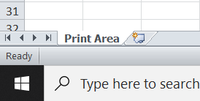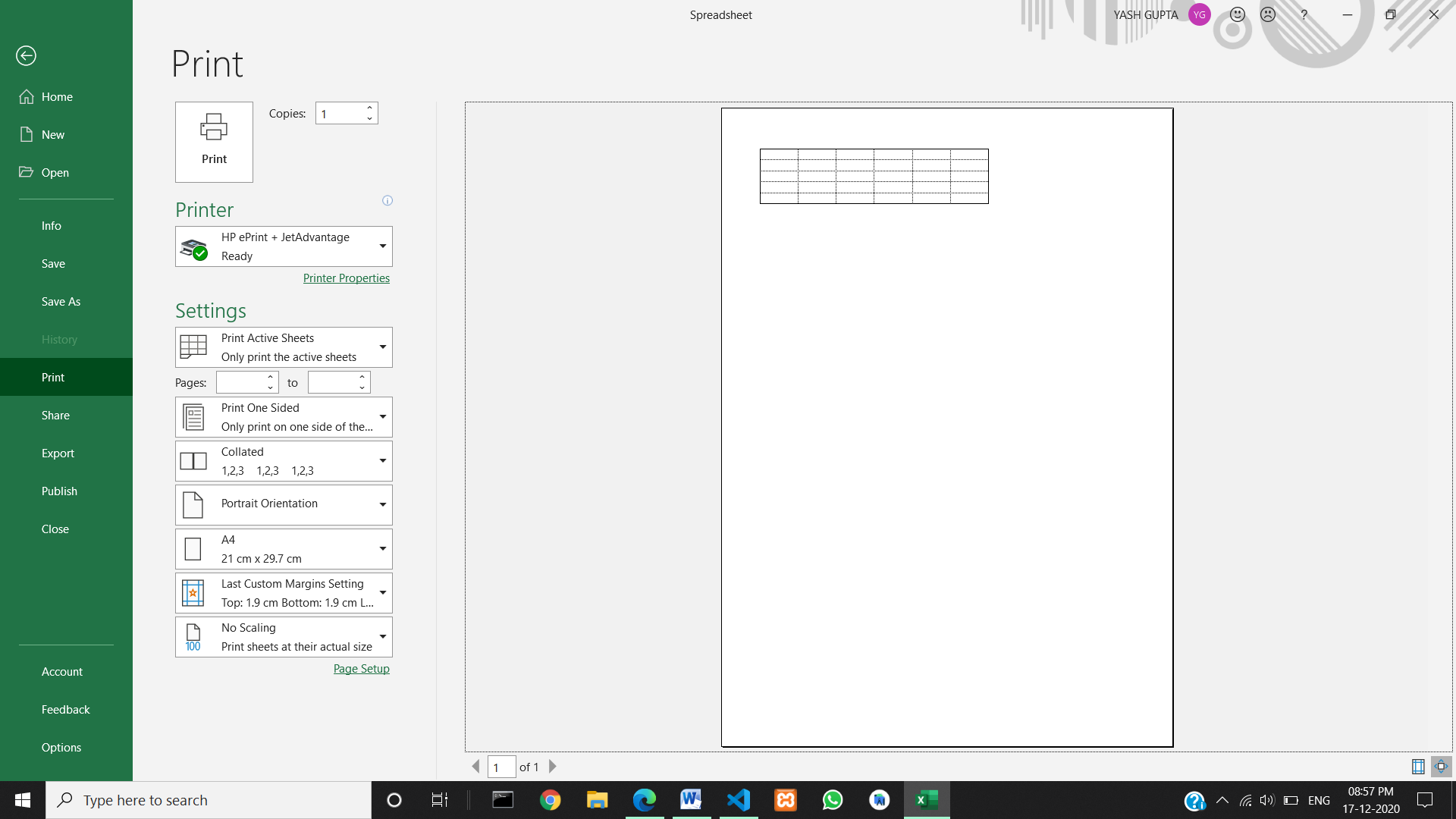# How to Set the Print Area of a Spreadsheet Using Java?

• Last Updated : 19 Jan, 2021

We can set the print area in a Spreadsheet. This can be done using the Apache POI library of Java. We use the different classes and methods of POI library to set a print area of the spreadsheet. Usually, it takes from top left to the bottom right of the Excel Spreadsheets. It can be customized as per the requirement. We can print a particular range of cells from the whole spreadsheet, customize the paper size, print the contents with the grid lines turned on, etc.

Create a new workbook

Attention reader! Don’t stop learning now. Get hold of all the important Java Foundation and Collections concepts with the Fundamentals of Java and Java Collections Course at a student-friendly price and become industry ready. To complete your preparation from learning a language to DS Algo and many more,  please refer Complete Interview Preparation Course.

We can create a new workbook and a spreadsheet using the various POI classes. First, we have to create a workbook then we create a spreadsheet in that workbook. Here we create a workbook object named ‘workbook’ using XSSFWorkBook class. Then we created a spreadsheet named as ‘Print Area’ using the createSheet() function of XSSFSheet class.

To create the workbook:

```// Create a Work Book
XSSFWorkbook workbook = new XSSFWorkbook();```

To create the spreadsheet in that workbook:

```// Create spreadsheet named "Print Area"

Setup of Print Area

Now we set up the print area using the setPrintArea() function. In this function, we send the five values.

```workbook.setPrintArea(
0, // sheet index
0, // start column
5, // end column
0, // start row
5 // end row
);```

We can display the grid lines in the print area by using the setDisplayGridlines() function.

```// set display grid lines or not

To set the paper size, we use the setPaperSize() function.

```// set paper size

If we want to print the gridlines also with our content, we can do that by the setPrintGridlines() function.

```// set print grid lines or not

Below is the implementation of the problem statement:

## Java

 `// Java program to set the print area in spreadsheet`` ` `import` `java.io.*;``import` `org.apache.poi.xssf.usermodel.XSSFPrintSetup;``import` `org.apache.poi.xssf.usermodel.XSSFSheet;``import` `org.apache.poi.xssf.usermodel.XSSFWorkbook;`` ` `public` `class` `GFG {``    ``public` `static` `void` `main(String[] args) ``throws` `Exception``    ``{`` ` `        ``// Create a Work Book``        ``XSSFWorkbook workbook = ``new` `XSSFWorkbook();`` ` `        ``// Create spreadsheet named "Print Area"``        ``XSSFSheet spreadsheet``            ``= workbook.createSheet(``"Print Area"``);`` ` `        ``// Set print area with indexes``        ``workbook.setPrintArea(``0``, ``// Sheet index``                              ``0``, ``// Start column``                              ``5``, ``// End column``                              ``0``, ``// Start row``                              ``5` `// End row``        ``);`` ` `        ``// Set display grid lines or not``        ``spreadsheet.setDisplayGridlines(``true``);`` ` `        ``// Set paper size``        ``spreadsheet.getPrintSetup().setPaperSize(``            ``XSSFPrintSetup.A4_PAPERSIZE);`` ` `        ``// Set print grid lines or not``        ``spreadsheet.setPrintGridlines(``true``);`` ` `        ``// Enter file path/name here``        ``FileOutputStream out = ``new` `FileOutputStream(``            ``new` `File(``"GFGSpreadsheet.xlsx"``));`` ` `        ``workbook.write(out);``        ``out.close();`` ` `        ``System.out.println(``            ``"GFGSpreadsheet.xlsx has been created"``);``    ``}``}`

Output:

After we compile the above program, it will create a ‘GFGSpreadsheet.xlsx’ file

in your computer. Then in this file, a workbook is created and in that workbook

a Spreadsheet named “Print Area” is created.Then we selected the rows and columns we have to print and some setups we applied to the print area.

Then after all that got executed we get the following confirmation line as mentioned in the code.

`GFGSpreadsheet.xlsx has been created`

As in our code, we have not set any values for the cells, so the file is a blank file. In the print preview tab, the cells we have selected for printing will be empty. Although as per our code, we have written the code to display the gridlines in the print area.

Final Output of our code shown below:My Personal Notes arrow_drop_up